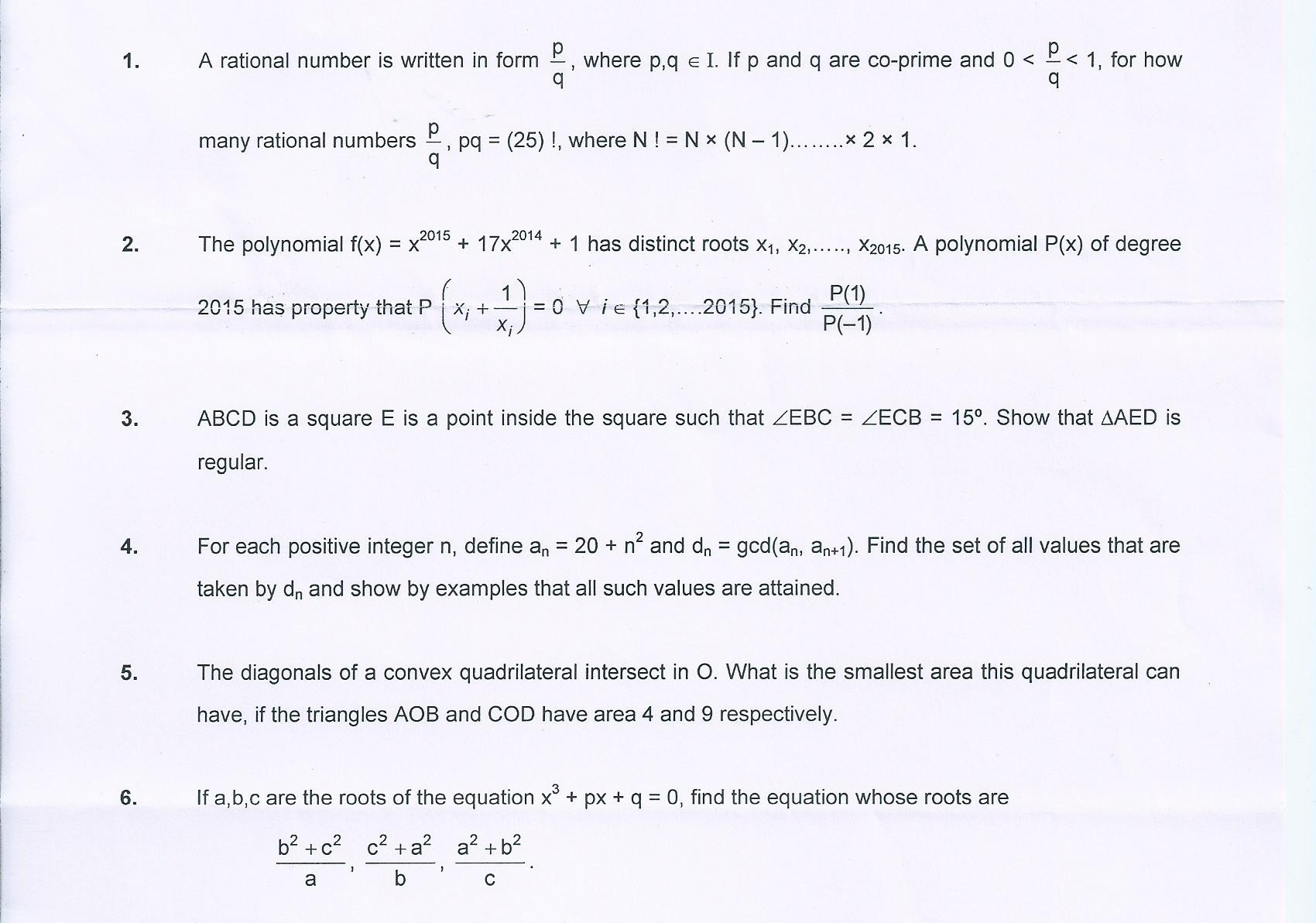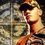# Olympiad preparation begins ...hereHere are some Olympiad preparatory problems. Please share your views on the problems and spread them by resharing ..

(Solutions are invited)Note by Kislay Raj
6 years, 9 months ago

This discussion board is a place to discuss our Daily Challenges and the math and science related to those challenges. Explanations are more than just a solution — they should explain the steps and thinking strategies that you used to obtain the solution. Comments should further the discussion of math and science.

When posting on Brilliant:

• Use the emojis to react to an explanation, whether you're congratulating a job well done , or just really confused .
• Ask specific questions about the challenge or the steps in somebody's explanation. Well-posed questions can add a lot to the discussion, but posting "I don't understand!" doesn't help anyone.
• Try to contribute something new to the discussion, whether it is an extension, generalization or other idea related to the challenge.
• Stay on topic — we're all here to learn more about math and science, not to hear about your favorite get-rich-quick scheme or current world events.

MarkdownAppears as
*italics* or _italics_ italics
**bold** or __bold__ bold
- bulleted- list
• bulleted
• list
1. numbered2. list
1. numbered
2. list
Note: you must add a full line of space before and after lists for them to show up correctly
paragraph 1paragraph 2

paragraph 1

paragraph 2

[example link](https://brilliant.org)example link
> This is a quote
This is a quote
    # I indented these lines
# 4 spaces, and now they show
# up as a code block.

print "hello world"
# I indented these lines
# 4 spaces, and now they show
# up as a code block.

print "hello world"
MathAppears as
Remember to wrap math in $$ ... $$ or $ ... $ to ensure proper formatting.
2 \times 3 $2 \times 3$
2^{34} $2^{34}$
a_{i-1} $a_{i-1}$
\frac{2}{3} $\frac{2}{3}$
\sqrt{2} $\sqrt{2}$
\sum_{i=1}^3 $\sum_{i=1}^3$
\sin \theta $\sin \theta$
\boxed{123} $\boxed{123}$

## Comments

Sort by:

Top Newest

No offense, but #6 is super ugly and bashy... I got

$x^3-\frac{2p^2}{q}x^2-5px+\frac{2p^3-q^2}q$

- 6 years, 9 months ago

Log in to reply

To this I say #NoBash. :P

- 6 years, 9 months ago

Log in to reply

no.6 was designed by the overlord of hell.

- 6 years, 9 months ago

Log in to reply

hey cody ....yeah u are right ..#6 was a long one.....what do u think of the other problems...?

- 6 years, 9 months ago

Log in to reply

For #1,

We know that $25! = 2^{a_1} \times 3^{a_2} \times 5^{a_3} \times \dots \times 23^{a_9}$ for some natural numbers $a_1,a_2,\ldots, a_9$.

Since $p$ and $q$ are coprime and $pq =25!$, if $m_n|q$ for some prime $m_n$, then $m_n^{a_n}|p$. Therefore, the factors of $p$ are from the set $[2^{a_1},3^{a_2},5^{a_3},\dots, 23^{a_9}]$.Since there are two possible choices for each of the 9 factors(i.e either $m_n^{a_n}|p$ or $m_n^{a_n}\not|p )$ there are $2^9$ possible values of $p$ and therefore $2^9$ values of $\frac{p}{q}$

Now we have to find how many of these values satisfy $0 < \frac{p}{q} < 1$.The first half of the inequality is trivially true for all pairs. Now for every fraction $\frac{p_i}{q_i}$, there exists a fraction $\frac{p_j}{q_j}$ such that $p_i = q_j$ and $q_i = p_j$. Now, since $\dfrac{p_i}{q_i} \times \dfrac{p_j}{q_j} = \dfrac{p_i}{q_i} \times \dfrac{q_i}{p_i} = 1$ and $\frac{p_i}{q_i} \not = 1$ for all $i$, it implies that for each pair of fractions $\frac{p_i}{q_i}$ and $\frac{p_j}{q_j}$, one fraction is > 1 and the other < 1. Since there are $\frac{2^9}{2} = 2^8$ pairs of fractions, there are $\boxed{2^8 = 256}$ fractions which satisfy the given conditions

- 6 years, 9 months ago

Log in to reply

wow ...a very good solution .. i think u cracked the toughest part :)

- 6 years, 9 months ago

Log in to reply

Sol #3 (Recommend to draw with me.) (All these numbers and variables are in degrees.)

Midpoint of $\overline{AD}, \overline{BC}$ is $F,G$ respectively. Diagonal of the square meets at $H$. Draw $\overline{AE}$ bisects $\overline{BD}$ at $I$.

Let $A\hat{E}H = a, E\hat{A}H = b, A\hat{E}B = c$.

We know that $A\hat{H}F = 45$, $B\hat{E}G = 75$, $E\hat{A}B = 45 - b$, $A\hat{I}B = c+30$

$\triangle AEH; a + b = 45$

Straight angle at $E; a + c + 75 = 180$

$\triangle EAB; 45-b+c+30+75 = 180$

Solve three equations we get $a = 30, b = 15, c = 75$.

Since $E\hat{A}D = 45 + b = 60$, by symmetry, $E\hat{D}A = 60$.

Therefore, $\triangle AED$ is equilateral triangle. Meow!

If someone have better solution without solving equations, that'd be great! ^__^

- 6 years, 9 months ago

Log in to reply

There exist a very easy solution to this problem I will add the diagram, can somebody tell me how to link images here? I put this text, but apparently it doesn't work.$![alt text](/Users/JordiBosch/Desktop/TDR/DEMO.png "Title")$

- 6 years, 9 months ago

Log in to reply

I haven't done this before, but you should upload this img into website instead. Sorry I don't know the website =="

- 6 years, 9 months ago

Log in to reply

If you can't do that, you can just tell everyone how to draw that. I know everyone can.

- 6 years, 9 months ago

Log in to reply

hey Samuraiwarm Tsunayoshi, yor solution is really a good and short one

- 6 years, 9 months ago

Log in to reply

Isn't this Resonance opt paper?

- 6 years, 9 months ago

Log in to reply

(2) is interesting. The answer seems to be $73/64$. If the polynomial is $x^n + 17x^{n-1} + 1$, the answer only depends on $n$ mod $6$. I have most of a proof but will have to wait until tomorrow to write it up.

- 6 years, 9 months ago

Log in to reply

sol #4.
we have to find gcd of 20+ $n^{2}$ and20+ $(n+1)^{2}$ .
which is the same as gcd(20+ $n^{2}$ , 20+ $n^{2}$+2n+1).
which is the same as gcd(20+ $n^{2}$ , 2n+1).
let 2n+1=p ==> n=(p-1)/2 we have to find gcd(20+($(p-1)^{2}$/4) , p).
opening p-1 square we have gcd( (81+$p^{2}$-2p)/4 ,p).
for 81+$p^{2}$-2p to share a factor with p, p is power of three.
clearly possible gcds are 1,3,9,27and 81(all powers of three not greater than 81)

- 6 years, 9 months ago

Log in to reply

sol #5.
let angleAOB=X.
thus COD =X .
BOC=180-X.
AOD=180-X.
let AO=a, BO=b, CO=c, DO=d.
ab sinX=4. (area of AOB). eq1.
cd sinx=9. (area of COD). eq2.
area of triangle BOC= bd sin(180-X)= bd sinX.
similarly area(AOD)=ac sinX.
area(quadrilateral)= 4+9+ bd sinX+ac sinX.
=13+sinX(ac+bd).
(from eq2 sinX=9/cd).
=13+9/cd(ac+bd).
=13+9(b/c+a/d).
from am gm inequality b/c+a/d>=2sqrt(ab/cd)=4/3.
therefore min =13+9(4/3).
=25.

- 6 years, 9 months ago

Log in to reply

For (4), $d_n = 1,3,9,27,81$ are the possibilities. These are attained at $n = 2, 1, 4, 13, 40$ respectively. To see that these are the only ones, note that if $d | a_n$ and $d | a_{n+1}$, then $d | (4a_n - (2n-1)(a_{n+1}-a_n))$, and $4a_n - (2n-1)(a_{n+1}-a_n)$ happens to equal $81$.

- 6 years, 9 months ago

Log in to reply

I think that 1,4 are very well known problems. First has appeared in RMO too

- 6 years, 9 months ago

Log in to reply

Sorry, I MEANT 1,3

- 6 years, 9 months ago

Log in to reply

×

Problem Loading...

Note Loading...

Set Loading...